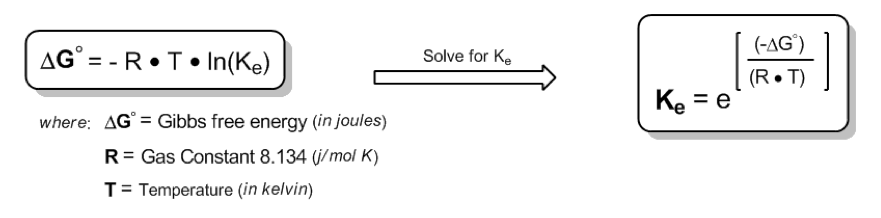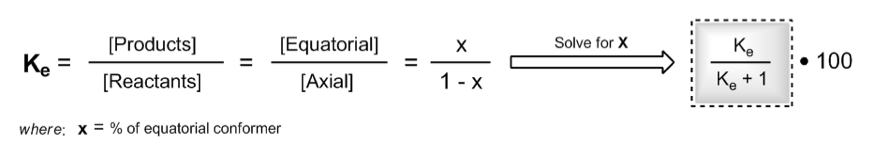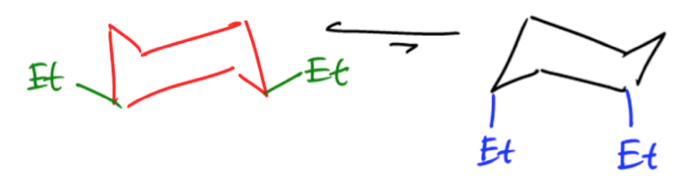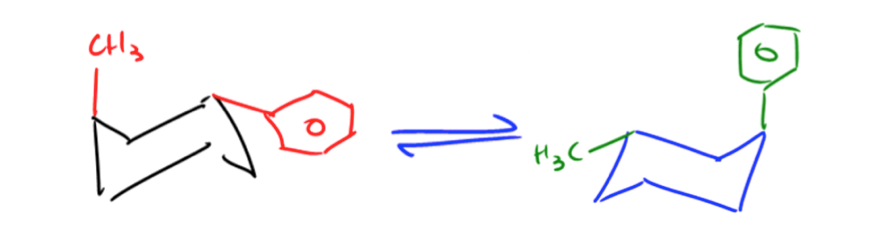Ch. 4 - Alkanes and CycloalkanesWorksheetSee all chapters
 Ch. 1 - A Review of General Chemistry 4hrs & 47mins 0% complete Worksheet Ch. 2 - Molecular Representations 1hr & 12mins 0% complete Worksheet Ch. 3 - Acids and Bases 2hrs & 45mins 0% complete Worksheet Ch. 4 - Alkanes and Cycloalkanes 4hrs & 18mins 0% complete Worksheet Ch. 5 - Chirality 3hrs & 33mins 0% complete Worksheet Ch. 6 - Thermodynamics and Kinetics 1hr & 19mins 0% complete Worksheet Ch. 7 - Substitution Reactions 1hr & 46mins 0% complete Worksheet Ch. 8 - Elimination Reactions 2hrs & 24mins 0% complete Worksheet Ch. 9 - Alkenes and Alkynes 2hrs & 10mins 0% complete Worksheet Ch. 10 - Addition Reactions 3hrs & 33mins 0% complete Worksheet Ch. 11 - Radical Reactions 1hr & 57mins 0% complete Worksheet Ch. 12 - Alcohols, Ethers, Epoxides and Thiols 2hrs & 34mins 0% complete Worksheet Ch. 13 - Alcohols and Carbonyl Compounds 2hrs & 14mins 0% complete Worksheet Ch. 14 - Synthetic Techniques 1hr & 28mins 0% complete Worksheet Ch. 15 - Analytical Techniques: IR, NMR, Mass Spect 7hrs & 18mins 0% complete Worksheet Ch. 16 - Conjugated Systems 5hrs & 49mins 0% complete Worksheet Ch. 17 - Aromaticity 2hrs & 24mins 0% complete Worksheet Ch. 18 - Reactions of Aromatics: EAS and Beyond 4hrs & 31mins 0% complete Worksheet Ch. 19 - Aldehydes and Ketones: Nucleophilic Addition 4hrs & 54mins 0% complete Worksheet Ch. 20 - Carboxylic Acid Derivatives: NAS 2hrs & 3mins 0% complete Worksheet Ch. 21 - Enolate Chemistry: Reactions at the Alpha-Carbon 1hr & 59mins 0% complete Worksheet Ch. 22 - Condensation Chemistry 2hrs & 13mins 0% complete Worksheet Ch. 23 - Amines 1hr & 43mins 0% complete Worksheet Ch. 24 - Carbohydrates 5hrs & 56mins 0% complete Worksheet Ch. 25 - Phenols 15mins 0% complete Worksheet Ch. 26 - Amino Acids, Peptides, and Proteins 2hrs & 54mins 0% complete Worksheet

# A-Values

See all sections
Sections
IUPAC Naming
Alkyl Groups
Naming Cycloalkanes
Naming Bicyclic Compounds
Naming Alkyl Halides
Naming Alkenes
Naming Alcohols
Naming Amines
Cis vs Trans
Conformational Isomers
Newman Projections
Drawing Newman Projections
Barrier To Rotation
Ring Strain
Axial vs Equatorial
Cis vs Trans Conformations
Equatorial Preference
Chair Flip
Calculating Energy Difference Between Chair Conformations
A-Values
Decalin
Alkyl
t-Butyl, sec-Butyl, isobutyl, n-butyl

Now that we know how to calculate the difference in flip energy, we can plug that information into the famous Equilibrium Constant equation to determine exact Ke of the reaction.

Concept #1: Calculate the difference in Gibbs free energy between the alternative chair conformations of trans-4-iodo-1-cyclohexanol.

Transcript

All right guys, so in these videos I'm going to teach you how to use free energy in kilojoules per mole to calculate the exact percentages of each conformation that you would get of a cyclohexane. Again, these videos might be beyond the scope of what your professor wants you to know for your test, so I'm going to leave it up to you to decide if you need to know this or not. So you've been warned, so it's time to get into this.
It turns out that we can use that delta G value that we get from our A values to calculate those exact percentages at any given temperature. Now the way we do this is through the Gibbs free energy equilibrium constant equation. Just so you guys know, if this equation looks familiar, it's not unique to this type of problem. In fact, pretty much any process that you can describe a free energy difference in can be – you can determine an equilibrium through this equation. This is a very important equation for all of chemistry, not just for cyclohexanes.
As you can see, what it says is that – let's just go through one term at a time. It says that the delta G or the change in free energy is equal to the negative R. Now remember that R is the gas constant that we used to use in general chemistry. And there were two different values of R that we used to use. Well, just note that the one we're using is in joules per mole so that's 8.134. That's going to be important in a second.
Then temperature. Temperature is in Kelvin. Remember that – it's been a while since we've dealt with temperature, but remember that 0 degrees Celcius is equal to 273.15 degrees Kelvin. That's going to be a conversion that we have to use in a little bit.
Then you're going to multiply that by the natural log of the equilibrium constant. Well, in order to solve any of these problems for percentages, we need to know the value of the equilibrium constant because equilibrium constant, by definition, tells you what's your products over your reactants. I need to know that fraction.
If we go ahead and we solve for Ke – I did the math for you. Don't worry. What we get is that the Ke is equal to the negative delta G over the R times the T all to the e. If we can just plug in these variables, we're going to get the equilibrium constant. Now we know what R is. We know what T is. Your calculator tells you what e is. All we need is negative delta G. Do we have a way to find that? Yes, guys, that's through our A values. Our A values tell us what the free energy change is as we go axial. All right? Awesome.
Now I do want to make one note of the delta G. Notice that this is negative delta G, but everything that we solved when we were doing A values, we were actually solving for positive delta G because we were actually looking at the less stable one. We were looking at how much energy do I have to put into the system to go to axial. So when we use this equation, we're actually going to be inputting the positive delta G here and that's fine. What we're going to be getting is a number that describes basically how we're going to that less stable value.
So then over here what we have is that – then we get that Ke and now we can solve for the percentages using the definition products over reactants. Now once we get that positive Ke number, that positive Ke number means that we're actually going toward the favored direction. I'm not sure if you guys remember, but if you have a Ke over 1, that means you're going to the more favored direction.
I'm just telling you guys right now, if we use a positive number for delta G, we're going to get also a positive number for – I'm sorry, we're going to get a number that's above 1 for Ke. We're going to get this greater than 1. It's going to be equal greater than 1. What that means is that our definition of Ke has to be the products over the reactants meaning the more favored conformation over the less favored.
Just letting us know that the way we've arranged this equation, the way that your textbook does it, is that it always does the more favored over the less favored, meaning that when you get this positive value, it's going to tell you what percentage you're going to have of the equatorial. Then that minus 100 will be your axial.
Here it says that Ke is equal to x over 1 over x. Guys, that just has to do with the definition of equilibrium constant, how x is what you're making. That's your product, so then 1 minus whatever you made would be your reactants.
Then we don't really want Ke here. We want x because we're really trying to figure out how much of this product we're going to get. If we solve for x, I did that for you, what you're finally going to get is that x is equal to Ke over Ke plus 1. And if you want to put it in a percentage term, it's times 100.
Now that was a tons of words and I just said a lot of numbers and symbols. I do not need you guys to perfectly understand this as much as I just need you to memorize it and know how to use it. If your professor wants you to solve this on your exam, then these equations should be in your mind. You should have memorized this equation. You should have memorized the definition of Ke or how to solve for x.
Now we're going to focus on the actual working part, on the actual part that we determine percentages, which is so cool. I'm a huge orgo nerd as you guys know, so this is fun. Getting to determine the exact percentage of each cyclohexane. This first one will be a worked example. We'll go ahead and start off with this first one. So I'm just going to pause the video and then we'll come back and we'll solve this one together.Gas Constant correct number: 8.314

Once we have the Ke of the equilibria, we can solve for x, which will be the percentage of my most favored chair.Practice: Estimate the equilibrium composition of the chair conformers of cis-1,3-diethylcyclohexane at room temperature.

Did you remember to use the correct Gas Constant number?! (8.314)Practice: Estimate the equilibrium composition of the chair conformers of trans-1-methyl-3-phenylcyclohexane at room temperature.Note: The correct value for methyl should be 7.6, not 4.2

With that, the correct answer should be closer to 88% / 12% for the percentage of both chairs using the correct value for R (8.314). Hope that makes sense!